# Samacheer Kalvi Books: Tamilnadu State Board Text Books Solutions

## Samacheer Kalvi 10th Science Chapter 10 Types of Chemical Reactions Notes PDF Download: Tamil Nadu STD 10th Science Chapter 10 Types of Chemical Reactions NotesSamacheer Kalvi 10th Science Chapter 10 Types of Chemical Reactions Notes PDF Download: Tamil Nadu STD 10th Science Chapter 10 Types of Chemical Reactions Notes

## Samacheer Kalvi 10th Science Chapter 10 Types of Chemical Reactions Notes PDF Download

We bring to you specially curated Samacheer Kalvi 10th Science Chapter 10 Types of Chemical Reactions Notes PDF which have been prepared by our subject experts after carefully following the trend of the exam in the last few years. The notes will not only serve for revision purposes, but also will have several cuts and easy methods to go about a difficult problem.

 Board Tamilnadu Board Study Material Notes Class Samacheer Kalvi 10th Science Subject 10th Science Chapter Chapter 10 Types of Chemical Reactions Format PDF Provider Samacheer Kalvi Books

## How to Download Samacheer Kalvi 10th Science Chapter 10 Types of Chemical Reactions Notes PDFs?

2. Click on the Samacheer Kalvi 10th Science Notes PDF.
3. Look for your preferred subject.
4. Now download the Samacheer Kalvi 10th Science Chapter 10 Types of Chemical Reactions notes PDF.

## Download Samacheer Kalvi 10th Science Chapter 10 Types of Chemical Reactions Chapterwise Notes PDF

### Samacheer Kalvi 10th Science Types of Chemical Reactions Textual Problems Solved

Chemical Reactions Questions And Answers Pdf Question 1.
Calculate the pH of 0.01 M HNO3?
Solution: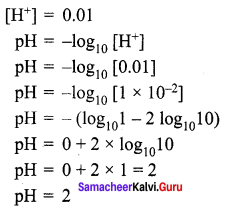Samacheer Kalvi Guru 10th Science Question 2.
The hydroxyl ion concentration of a solution is 1 × 10-9 M. What is the pOH of the solution?
Solution: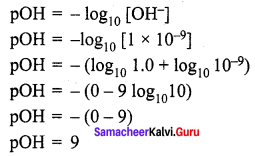Samacheer Kalvi Question 3.
A solution has a pOH of 11.76. What is the pH of this solution?
Solution:
pH = 14 – pOH
pH = 14 – 11.76 = 2.24.

10th Science Samacheer Kalvi Question 4.
Calculate the pH of 0.001 molar solution of HCl.
Solution:
HCl is a strong acid and is completely dissociated in its solutions according to the process:
HCl(aq)→H+(aq)+Cl−(aq)
From this process it is clear that one mole of HCl would give one mole of H+ ions.
Therefore, the concentration of H+ ions would be equal to that of HCl, i.e., 0.001 molar or 1.0 × 10-3 mol litre-1.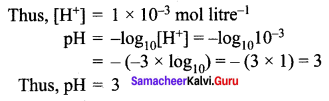Samacheer Kalvi 10th Science Question 5.
What would be the pH of an aqueous solution of sulphuric acid which is 5 × 10-5 mol litre-1 in concentration?
Solution:
Sulphuric acid dissociates in water as:
H2SO4(aq)→2H+(aq)+SO2−4(aq)
Each mole of sulphuric acid gives two moles of H+ ions in the solution. One litre of H2SO4 solution contains 5 × 10-5 moles of H2SO4 which would give 2 × 5 × 10-5 = 10 × 10-5 or 1.0 × 10-4 moles of H+ ion in one litre of the solution.
Therefore,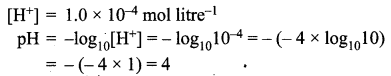10th Samacheer Kalvi Science Question 6.
Calculate the pH of 1 × 10-4 molar solution of NaOH.
Solution:
NaOH is a strong base and dissociates in its solution as:
NaOH(aq)→Na+(aq)+OH−(aq)
One mole of NaOH would give one mole of OH ions.
Therefore,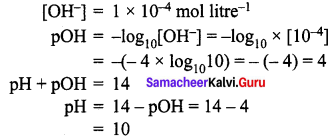10th Science Solution Samacheer Kalvi Question 7.
Calculate the pH of a solution in which the concentration of the hydrogen ions is 1.0 × 10-8 mol litre-1.
Solution:
Here, although the solution is extremely dilute, the concentration given is not of an acid or a base but that of H+ ions. Hence, the pH can be calculated from the relation: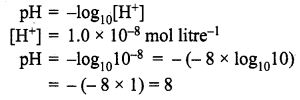Samacheer Kalvi 10th Science Solutions Question 8.
If the pH of a solution is 4.5, what is its pOH?
Solution:
pH + pOH = 14
⇒ pOH = 14 – 4.5 = 9.5
⇒ pOH = 9.5.

### Samacheer Kalvi 10th Science Types of Chemical Reactions Textual Evaluation Solved

Samacheer Kalvi 10th Science Solution Question 1.
H2(g)+Cl2(g)→2HCl(g) is a ______.
(a) Decomposition Reaction
(b) Combination Reaction
(c) Single Displacement Reaction
(d) Double Displacement Reaction.
(b) Combination Reaction
Hint: It is a combination reaction between H2 and Cl2 to form HCl as product.

Science Solution Class 10 Samacheer Kalvi Question 2.
Photolysis is a decomposition reaction caused by:
(a) heat
(b) electricity
(c) light
(d) mechanical energy
(c) light

Samacheer Kalvi 10 Science Question 3.
A reaction between carbon and oxygen is represented by C(s)+O2(g)→CO2(g) + Heat. In which of the type(s), the above reaction can be classified?
(i) Combination Reaction
(ii) Combustion Reaction
(iii) Decomposition Reaction
(iv) Irreversible Reaction
(a) i and ii
(b) i and iv
(c) i, ii and iii
(d) i, ii and iv.
(d) i, ii and iv.
Hint:

• It is a combination reaction with one product.
• It involves the combustion of carbon to form CO2.
• The reaction is irreversible and we cannot reverse the reaction.

Samacheer Kalvi Chemistry Question 4.
The chemical equation
Na2SO4(aq) + BaCI2(aq) → BaSO4(s)↓ + 2NaCl(aq) represents which of the following types of reaction?
(a) Neutralisation
(b) Combustion
(c) Precipitation
(d) Single displacement
(c) Precipitation

Samacheer Kalvi 10th Science Solution Book Question 5.
Which of the following statements are correct about a chemical equilibrium?
(i) It is dynamic in nature
(ii) The rate of the forward and backward reactions are equal at equilibrium
(iii) Irreversible reactions do not attain chemical equilibrium
(iv) The concentration of reactants and products may be different
(a) i, ii and iii
(b) i, ii and iv
(c) ii, iii and iv
(d) i, iii and iv.
(a) i, ii and iii
Hint: Chemical equilibrium is dynamic in nature for a reversible reaction.
At equilibrium, Rate of forwarding reaction = Rate of backward reaction.

Samacheer Kalvi Guru 10th Science Book Back Answers Question 6.
A single displacement reaction is represented by
X(s) + 2HCl(aq) → XCl2(aq) + H2(g). Which of the following(s) could be X?
(i) Zn
(ii) Ag
(iii) Cu
(iv) Mg. Choose the best pair.
(a) (i) and (ii)
(b) (ii) and (iii)
(c) (iii) and (iv)
(d) (i) and (iv)
(d) (i) and (iv)

Samacheer Kalvi 10th Standard Science Question 7.
Which of the following is not an “element + element → compound” type reaction?
(a) C(s)+O2(g)→CO2(g)
(b) 2K(s)+Br2(l)→2KBr(s)
(c) 2CO(g)+O2(g)→2CO2(g)
(d) 4Fe(s)+3O2(g)→2Fe2O3(s).
(c) 2CO(g)+O2(g)→2CO2(g)
Hint: It involves one reactant compound i.e., carbon monoxide (CO).
2CO(g)+O2(g)→2CO2

10th Standard Science Samacheer Kalvi Question 8.
Which of the following represents a precipitation reaction?
(a) A(s)+B(s)→C(s)+D(s)
(b) A(s)+B(aq)→C(aq)+D(l)
(c) A(aq)+B(aq)→C(s)+D(aq)
(d) A(aq)+B(s)→C(aq)+D(l).
(c) A(aq)+B(aq)→C(s)+D(aq)
Hint: It involves the formation of solid precipitation by mixing of two aqueous solutions.

10th Standard Samacheer Kalvi Science Question 9.
The pH of a solution is 3. Its [OH] concentration is:
(a) 1 × 10-3 M
(b) 3 M
(c) 1 × 10-11 M
(d) 11 M
(c) 1 × 10-11 M

Samacheer Kalvi 10th Science Book Question 10.
Powdered CaCO3 reacts more rapidly than flaky CaCO3 because of ______.
(a) large surface area
(b) high pressure
(c) high concentration
(d) high temperature.
(a) large surface area
Hint: We know that greater the surface area, faster will be a chemical reaction. Hence powdered CaCO3 reacts more rapidly.

II. Fill in the blanks.

Samacheer Kalvi Science 10th Question 1.
A reaction between an acid and a base is called ______.
Neutralization.

Question 2.
When lithium metal is placed in hydrochloric acid, _______ gas is evolved.
Hydrogen.

Question 3.
The equilibrium attained during the melting of ice is known as ______.
Physical equilibrium.

Question 4.
The pH of a fruit juice is 5.6. If you add slaked lime to this juice, its pH ______ (increase/decrease)
Increases.

Question 5.
The value of the ionic product of water at 25°C is ______.
1 × 10-14

Question 6.
The normal pH of human blood is ______.
7.4.

Question 7.
Electrolysis is type of _______ reaction.
Decomposition reaction.

Question 8.
The number of products formed in a synthesis reaction is ______.
One.

Question 9.
Chemical volcano is an example for ______ type of reaction.
Decomposition reaction.

Question 10.
The ion formed by dissolution of H+ in water is called ______.
Hydronium ion.

III. Match the following

Question 1.
Identify the types of reaction.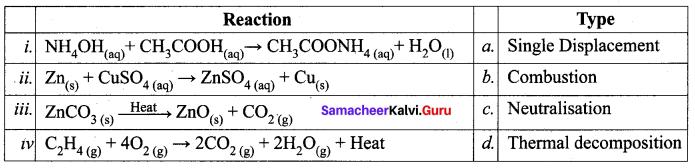i – c, ii-a, iii – d, iv- b.

IV. True or False: (If false give the correct statement)

Question 1.
Silver metal can displace hydrogen gas from nitric acid.
False.
Correct statement: In the activity series, any metals that are below hydrogen will not react with HNO3.

Question 2.
The pH of rainwater containing dissolved gases like SO3, CO2, NO2 will be less than 7.
True.

Question 3.
At the equilibrium of a reversible reaction, the concentration of the reactants and the products will be equal.
False.
Correct statement: At equilibrium rate of the forward reaction is equal to the rate of backward reaction.

Question 4.
Periodical removal of one of the products of a reversible reaction increases the yield.
True.

Question 5.
On dipping a pH paper in a solution, it turns into yellow. Then the solution is basic.
True.

Question 1.
When an aqueous solution of potassium chloride is added to an aqueous solution of silver nitrate, a white precipitate is formed. Give the chemical equation of this reaction.
KCl(aq)+AgNO3(aq)→AgCl(s)+KNO3(aq)
Formation of white precipitate by the above reaction is due to formation of silver chloride (AgCl).

Question 2.
Why does the reaction rate of a reaction increase on raising the temperature?
The rate of a reaction increases at higher temperature, because the heat to the reactants provide energy to breakup more bonds and speeds up the reaction.

Question 3.
Define a combination reaction. Give one example of an exothermic combination reaction.
A chemical reaction in which 2 or more reactants combine to form a single product, the reaction is known as combination reaction. Most of the combination reaction are exothermic because they involve formation of new bonds. For example,
H2(g)+Cl2(g)→2HCl(g)
SiO2(s)+CaO(s)→CaSiO3(s).

Question 4.
Differentiate reversible and irreversible reactions.

 Reversible reaction Irreversible reaction 1. Reactions can be reversed 1. The reaction cannot be reversed 2. It proceeds in both directions 2. It is unidirectional 3. It attains equilibrium 3. Equilibrium is not attained 4. It is relatively slow 4. It is fast

Question 1.
What is called thermolysis reactions?
A chemical reaction is a process in which old bond breaks up and new chemical bond get formed. Thermolysis chemical reactions is a special type of chemical reaction in which the reactant get decomposed by heat. For example,
CaCO3(s)⇌ Heat CaO(s)+CO2(g)
2HgO(s)⟶Heat2Hg(l)+O2(g)
In these reactions heat is supplied to break the bonds, so generally they are endothermic in nature.

Question 2.
Explain the types of double displacement reactions with examples.
When two compounds react, if their ions are interchanged, then the reaction is called double displacement reactions. There are two types of double displacement reactions. They are
(i) Precipitation reactions : When aqueous solutions of two compounds are mixed, if they react to form an insoluble compound and a soluble compound, then it is called precipitation reaction. Because the insoluble compound, formed as one of the products, is a precipitate and hence the reaction is so called.

(ii) When aqueous solutions of potassium iodide and lead (II) nitrate are mixed, a double displacement reaction takes place between them.
Pb(NO3)2(aq) + 2KI(aq) → PbI2(s)↓ + 2KNO3(aq).

(iii) Potassium and lead displace or replace one other and form a yellow precipitate of lead (II) iodide.

(iv) Neutralization reactions: When an acid reacts with the base to form a salt and water. It is called ‘neutralization reaction’ as both acid and base neutralize each other.

(v) Reaction of sodium hydroxide with hydrochloric acid is a typical neutralization reaction. Here, sodium replaces hydrogen from hydrochloric acid forming sodium chloride, a neutral soluble salt.
NaOH(aq) + HCl(aq) → NaCl(aq) + H2O(l)

Question 3.
Explain the factors influencing the rate of a reaction.
The factors influencing the rate of a reaction are,
(i) Nature of the reactants: The reaction of sodium with hydrochloric acid is faster than that with acetic acid. Do you know why? Hydrochloric acid is a stronger acid than acetic acid and thus more reactive. So, the nature of the reactants influences the reaction rate.
2Na(s)+2HCl(aq)2Na(s)+2CH3COOH(aq)→2NaCl(aq)+H2(g)(fast)→2CH3COONa(aq)+H2(g)(slow).

(ii) The concentration of the reactants: Changing the number of reactants also increases the reaction rate. The amount of the substance present in a certain volume of the solution is called ‘concentration’. More the concentration, more particles per volume exist in it and hence faster the reaction. Granulated zinc reacts faster with 2M hydrochloric acid than 1M hydrochloric acid.

(iii) Temperature: Most of the reactions go faster at a higher temperature. Because adding heat to the reactants provides energy to break more bonds and thus speed up the reaction. Calcium carbonate reacts slowly with hydrochloric acid at room temperature. When the reaction mixture is heated the reaction rate increases.

(iv) Pressure: If the reactants are gases, increasing their pressure increases the reaction rate. This is because on increasing the pressure the reacting particles come closer and collide frequently.

(v) Catalyst: A catalyst is a substance which increases the reaction rate without being consumed in the reaction. In certain reactions, adding a substance as catalyst speeds up the reaction. For example, on heating potassium chlorate, it decomposes into potassium chloride and oxygen gas, but at a slower rate. If manganese dioxide is added, it increases the reaction rate.

(vi) The surface area of the reactants: When solid reactants are involved in a reaction, their powdered form reacts more readily. For example, powdered calcium carbonate reacts more readily with hydrochloric acid than marble chips. Because powdering of the reactants increases the surface area and more energy is available on the collision of the reactant particles. Thus, the reaction rate is increased. You will study more about reaction rate in your higher classes.

Question 4.
How does pH play an important role in everyday life?
Our body works within the pH range of 7.0 to 7.8. Living organisms can survive only in a narrow range of pH change. Different body fluids have different pH values.
Eg: pH of blood is ranging from 7.35 to 7.45. Any increase or decrease in this value leads to diseases. The ideal pH for blood is 7.4.

pH in our digestive system : It is very interesting to note that our stomach produces hydrochloric acid. It helps in the digestion of food without harming the stomach. During indigestion the stomach produces too much acid and this causes pain and irritation. pH of the stomach fluid is approximately 2.0.

pH changes as the cause of tooth decay : pH of the saliva normally ranges between 6.5 to 7.5. White enamel coating of our teeth is calcium phosphate, the hardest substance in our body. When the pH of the mouth saliva falls below 5.5, the enamel gets weathered. Toothpastes, which are generally basic ate used for cleaning the teeth that can neutralise the excess acid and prevent tooth decay.

pH of soil : In agriculture, the pH of the soil is very important. Citrus fruits require slightly alkaline soil, while rice requires acidic soil and sugarcane requires neutral soil.

pH of rain water : The pH of rain water is approximately 7, which means that it is neutral and also represents its high purity. If the atmospheric air is polluted with oxide gases of sulphur and nitrogen, they get dissolved in the rain water and make its pH less than 7. Thus, if the pH of rain water is less than 7, then it is called acid rain. When acid rain flows into the rivers it lowers the pH of the river water also. The survival of aquatic life in such rivers becomes difficult.

Question 5.
What is chemical equilibrium? What are its characteristics?
Chemical equilibrium is the state for a reversible chemical reaction where the rate of forwarding direction is equally balanced by the rate of backward direction and the process seems like to be stopped.
Its characteristics are,

• The rate of the forward and backward reaction are equal in chemical equilibrium.
• The observable properties such as pressure, concentration, colour, density, viscosity, etc. of the system unchanged with time.
• In physical equilibrium, the volume of all phases remains constant.

VII. HOT Questions

Question 1.
A solid compound ‘A’ decomposes on heating into ‘B’ and a gas ‘C’ On passing the gas ‘C’ through water. It becomes acidic. Identify A, B and C.
CaCO3(s)⟶CaO(s)+CO2(g)
Calcium carbonate decomposes into solid Calcium oxide and a gas CO2. This CO2 dissolves in water and forms carbonic acid.
H2O+CO2⟶H2CO3 (Carbonic acid).

Question 2.
Can a nickel spatula be used to stir copper sulphate solution? Justify your answer.
No, a nickel spatula cannot be used to stir CuSO4 solution, because Nickel will displace copper from CuSO3 solution and Cu gets deposited on the Ni spatula.

VIII. Solve the following problems.

Question 1.
Lemon juice has a pH = 2, what is the concentration of H+ ions?
Solution: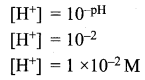Question 2.
Calculate the pH of 1.0 × 10-4 molar solution of HNO3?
Solution: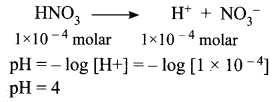Question 3.
What is the pH of 1.0 × 10-5 molar solution of KOH?
Solution: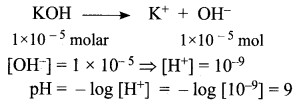Question 4.
The hydroxide ion concentration of a solution is 1 × 10-11. What is the pH of the solution?
Solution: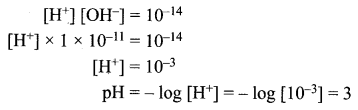### Samacheer Kalvi 10th Science Types of Chemical Reactions Additional Question Solved

Question 1.
Methane + Oxygen → A + Water. Identify A ______.
(a) Carbon monoxide
(b) Carbon dioxide
(c) ethane
(d) LPG.
(i) Carbon dioxide

Question 2.
The chemical reaction in which electricity is used to bring about the change is:
(a) Thermal decomposition
(b) Photo decomposition
(c) Single displacement reaction
(d) Electrolytic decomposition
(d) Electrolytic decomposition

Question 3.
Decomposition of the molecule occurs on passing an electric current through its aqueous solution. This process is termed as ______.
(a) Thermolysis
(b) Photolysis
(c) Electrolysis
(d) None of these.
(c) Electrolysis

Question 4.
The most reactive element in the activity series is:
(a) platinum
(b) potassium
(c) sodium
(d) gold
(b) potassium

Question 5.
Which one of the following is a more reactive element?
(a) Cu
(b) Li
(c) Zn
(d) Pb.
(b) Li

Question 6.
Which one of the following is the least reactive element?
(a) Au
(b) Fe
(c) Ca
(d) Na.
(a) Au

Question 7.
Double displacement reaction is also called as ______.
(a) Metastasis
(b) Metathesis
(c) Methanolysis
(d) Metalysis.
(b) Metathesis

Question 8.
The acidic solution among the following is:
(a) seawater
(b) coffee
(c) lime water
(d) antacid
(b) coffee

Question 9.
The reaction of sodium hydroxide with hydrochloric acid is an example for ______.
(a) Combination reaction
(b) Thermolysis reaction
(c) Neutralization reaction
(d) Precipitation reaction.
(c) Neutralization reaction

Question 10.
In agriculture, the nature of the soil for rice is:
(a) alkaline
(b) neutral
(c) acidic
(d) none of the above
(c) acidic

Question 11.
The role of manganese dioxide in the decomposition of potassium chlorate is ______.
(a) act as a reactant
(b) act as a catalyst
(c) act as a reagent
(d) act as a reaction medium.
(b) act as a catalyst

Question 12.
The ionic product of water Kw is ______.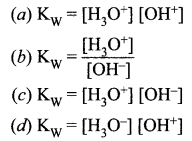(c) KW=[H3O+][OH−]

Question 13.
Unit of ionic product of water is ______.
(a) mol-2 dm-6
(b) mol2 dm-6
(c) mol2 dm6
(d) mol dm-3.
(b) mol2 dm-6

Question 14.
What is the value of ionic product of water?
(a) 1 × 10-4
(b) 1 × 10-1
(c) 1 × 1014
(d) 1 × 10-14.
(d) 1 × 10-14.

Question 15.
pH of milk of magnesia is ______.
(a) 10
(b) 15
(c) 14
(d) 10.5.
(a) 10

Question 16.
What is the relationship between pH and pOH?
(a) pH – pOH = 14
(b) pH + pOH = 14
(c) pH/pOH = 14
(d) pH + pOH = 1.4.
(b) pH + pOH = 14

Question 17.
Zn+2HCl→ZnCl2+H2↑
The above reaction is an example of ______.
(а) Combination reaction
(b) Double displacement reaction
(c) Displacement reaction
(d) Decomposition reaction.
(c) Displacement reaction

Question 18.
A student tests the pH of pure water using a pH paper. It shows a green colour. If a pH paper is after adding lemon juice to water, what colour will he observe?
(a) Green
(b) Red
(c) Yellow
(d) Pink.
(b) Red

Question 19.
When aqueous solution of silver nitrate and sodium chloride are mixed, ______ precipitate is immediately formed.
(a) white
(b) yellow
(c) red
(d) black.
(a) white

Question 20.
pH = – log10 [H+]. The pH of a solution containing hydrogen ion concentration of 0.001 M solution is ______.
(a) 3
(b) 11
(c) 14
(d) 15.
(a) 3

Question 21.
Silver anklet has got tarnished due to the formation of ______.
(a) Ag2O
(b) AgNO3
(c) Ag2S
(d) AgBr.
(c) Ag2S

Question 22.
The colour of the precipitate formed by the reaction of lead nitrate with potassium iodide is ______.
(a) white
(b) yellow
(c) black
(d) red.
(b) yellow

Question 23.
The reaction of calcium oxide with water is a ______ reaction.
(a) exothermic
(b) endothermic
(c) isothermic
(a) exothermic

Question 24.
The gas released when calcium carbonate reacts with dilute hydrochloric acid is ______.
(a) NO2
(b) CO
(c) CO2
(d) H2.
(c) CO2

Question 25.
The chemical used in whitewashing on the walls is ______.
(a) CaO
(b) CaCl2
(c) CaOCl2
(d) Ca(OH)2.
(d) Ca(OH)2.

Question 26.
The chemical formula of marble is ______.
(a) CaCO3
(b) CaOCl2
(c) CaSO4. 12 H2O
(d) Ca(OH)2.
(a) CaCO3

Question 27.
Combustion of coal is an example of _____ reaction.
(a) displacement
(b) reduction
(c) decomposition
(d) combination.
(d) combination.

Question 28.
The colour change takes place when copper carbonate is strongly heated is ______.
(a) green to black
(b) green to blue
(c) blue to green
(d) blue to black.
(a) green to black

Question 29.
The reaction of heat on copper carbonate into copper (II) oxide is _________ reaction.
(a) combination
(b) decomposition
(c) displacement
(d) redox.
(b) decomposition

Question 30.
The dissolution of glucose in water is a _____ reaction.
(a) exothermic
(b) endothermic
(c) neutralisation
(d) combination.
(b) endothermic

Question 31.
All combustion reactions are _______ reactions.
(a) combination
(b) exothermic
(c) endothermic
(d) neutralisation.
(b) exothermic

Question 32.
The factor that affects the rate of the chemical reaction is ______.
(a) temperature
(b) concentration
(c) catalyst
(d) all the above.
(d) all the above.

Question 33.
Magnesium ribbon reacts at a very faster rate with ______ acid.
(a) hydrochloric
(b) acetic
(c) formic
(d) oxalic.
(a) hydrochloric

Question 34.
Our body metabolism is carried out by means of ______ secreted in our stomach.
(a) sulphuric acid
(b) hydrochloric acid
(c) nitric acid
(d) acetic acid.
(b) hydrochloric acid

Question 35.
Which metals do not liberate gas on reaction with acids?
(a) Zn, Mg
(b) Ag, Cu
(c) Na, K
(d) Cr, Al.
(b) Ag, Cu

Question 36.
When CO2 is passed through lime water, it turns ______.
(a) milky
(b) black
(c) red
(d) blue.
(a) milky

Question 37.
The physical form of calcium carbonate is ______.
(a) limestone
(b) chalk
(c) marble
(d) all the above.
(d) all the above.

Question 38.
The colour change takes place when copper (II) oxide reacts with dilute hydrochloric acid is ______.
(a) blue to green
(b) black to green
(c) green to black
(d) green to blue.
(b) black to green

Question 39.
Which of the following is a strong base?
(a) NH4OH
(b) Ca(OH)2
(c) Al(OH)3
(d) NaOH.
(d) NaOH.

Question 40.
Zinc reacts with sodium hydroxide to form ______.
(a) Zinc hydroxide + H2O
(b) Sodium zincate + H2
(c) Zinc oxide + H2
(d) Zinc oxide + H2O.
(b) Sodium zincate + H2

Question 41.
_____ reacts with sodium hydroxide.
(a) Cu
(b) Ag
(c) Cr
(d) Zn.
(d) Zn.

Question 42.
________ is used as a medicine for stomach disorder.
(a) Sodium hydroxide
(b) Ammonium hydroxide
(c) Magnesium hydroxide
(d) Calcium hydroxide.
(c) Magnesium hydroxide

Question 43.
The pH of stomach fluid is ______.
(a) 12
(b) 14
(c) 2
(d) 1.
(c) 2

Question 42.
The hardest substance in the human body is ______.
(a) bone
(b) the enamel coating of teeth
(c) brain
(d) liver.
(b) the enamel coating of teeth

Question 43.
Sugarcane requires _______ soil.
(a) acidic
(b) alkaline
(c) neutral
(d) amphoteric.
(c) neutral

Question 44.
Rice requires _____ soil.
(a) acidic
(b) basic
(c) alkaline
(d) neutral.
(a) acidic

Question 45.
______ is a double salt.
(a) Sodium chloride
(b) Washing soda
(c) Potash alum
(d) Bleaching powder.
(c) Potash alum

II. Fill in the blanks.

Question 1.
During chemical changes _____ are formed and these changes are more _____ than physical changes.
New products, permanent.

Question 2.
Calcium oxide reacts with water to produce ______ and the reaction is ______.
Slaked lime, exothermic.

Question 3.
During whitewashing, ______ reacts slowly with carbon dioxide in the air to form a thin layer of _______ on the walls.
Calcium hydroxide, calcium carbonate.

Question 4.
When copper carbonate is heated, the products formed are _____ , ______ and change of colour from ______ to ____ is observed.
CuO, CO2, green, black

Question 5.
When lead nitrate is heated, the gas liberated is ______ and its colour is ______.
NO2, Reddish – brown

Question 6.
Copper sulphate solution changes its blue colour into _____ colour when an iron nail is added to it and it acquires _____ colour.
Green, brownish.

Question 7.
When Barium chloride reacts with sodium sulphate, the product formed is _____ and it is a ______ precipitate.
Barium sulphate, white.

Question 8.
Powdered ____ reacts more quickly with hydrochloric acid than marble chips.
CaCO3

Question 9.
Calcium carbonate present in the marble reacts with hydrochloric acid at a faster rate at _______ temperature.
Higher.

Question 10.
______ is a substance which furnishes H+ ions when dissolved in water and a ______ is a substance which furnishes OH ions in water.
Acid, Base.

Question 11.
Acids present in plants and animals are ______ and the acids in rocks and minerals are ______.
Organic acid, inorganic acid.

Question 12.
Metal displaces _______ gas from dilute acid and the flame goes off with a ______ sound.
Hydrogen, popping.

Question 13.
_____ is used in the manufacturing of soap and ______ is used in whitewashing buildings.
Sodium hydroxide, calcium hydroxide.

Question 14.
pH scale was introduced by ______ and the pH of the solution is 7, it is a ______ solution.
S.P.L. Sorenson, neutral.

Question 15.
The pH of a normal healthy human skin is _____ and the pH of the stomach fluid is ______.
7.4, 5.5.

Question 16.
The ideal pH for blood is _______ and pH of the mouth falls below ______.
7.35 – 7.45, Sulphuric acid.

Question 17.
All photo decomposition reaction are _______ reactions.
Endothermic.

Question 18.
Precipitation reactions give a _____ as the product.
Insoluble salt.

Question 19.
Plants cannot grow in a ______ soil.
Acidic.

Question 20.
Equilibrium is possible in a _____ system.
Closed.

Question 21.
Pure water is a _______ electrolyte.
Weak.

Question 22.
Most of the combination reactions are _______ in nature.
Exothermic.

Question 23.
Silicon dioxide reacts with calcium oxide to form ______.
Calcium silicate.

Question 24.
Our mobile phones get energy from its _____ battery by chemical reaction.
Lithium.

Question 25.
A ______ is a substance which increases the reaction rate.
Catalyst.

Question 26.
pH range of human saliva is ______.
6 – 8.

Question 27.
pH range of fresh milk is ______.
5.

Question 28.
The pH of a solution can be determined by using a _______ indicator.
Universal.

Question 29.
pH is ______.
-log10 [H+].

Question 30.
The white enamel coating of our teeth is ______.
Calcium phosphate.

III. Match the following.

Question 1.

 (i) S(s)+O2(g)→SO2(g) (a) Electrolytic decomposition reaction (ii) 2NaCl(aq)→2Na(s)+Cl2(g) (b) Combination reaction (iii) 2AgBr(s)→2Ag(s)+Br2(g) (c) Single displacement reaction (iv) Zn(s)+2HCl(aq)→ZnCl2(aq)+H2(g) (d) Photo decomposition reaction

i – b, ii – a, iii – d, iv – c.

Question 2.

 Sample pH (i) Oranges (a) 8 (ii) Grapes (b) 7 (iii) Pure water (c) 3.5 (iv) Egg white (d) 4

i – c, ii – d, iii – b, iv – a.

Question 3.

 Sample pH (i) Sour milk (a) 4.2 (ii) Tomato juice (b) 4.5 (iii) Seawater (c) 12 (iv) Lime water (d) 8

i – b, ii – a, iii – d, iv – c.

Question 4.

 (i) Citrus fruits (a) Neutral soil (ii) Rice (b) pH = 7 (iii) Sugarcane (c) alkaline soil (iv) Rainwater (d) acidic soil

i – c, ii – d, iii – a, iv – b.

Question 5.

 (i) Sodium hydroxide (a) medicine (ii) Calcium hydroxide (b) stain remover (iii) Ammonium hydroxide (c) soap (iv) Magnesium hydroxide (d) whitewashing

i – c, ii – d, iii – b, iv – a.

Question 6.

 (i) Gain of electrons (a) Oxidation reaction (ii) Loss of electrons (b) Combination reaction (iii) Combustion of coal (c) Redox reaction (iv) Rusting of iron (d) Reduction reaction

i – d, ii-a, iii – b, iv – e.

IV. State true or false. If false, give the correct statement.

Question 1.
Chemical changes are reversible changes.
False.
Correct statement: Chemical changes are irreversible changes.

Question 2.
The silver anklet has got tarnished when exposed to air due to the formation of silver oxide. (Ag2O).
False.
Correct statement: The silver anklet has got tarnished when exposed to air due to the formation of silver sulphide (Ag2S).

Question 3.
Brisk effervescence takes place with the evolution of CO2 when calcium carbonate reacts with dilute hydrochloric acid.
True.

Question 4.
The chemical formula for marble is CaCl2.
False.
Correct statement: The chemical formula for marble is CaCO3

Question 5.
A reaction in which a single product is formed from two or more reactants is known as displacement reaction.
False.
Correct statement: A reaction in which a single product is formed from two or more reactants is known as a combination reaction.

Question 6.
Lead Nitrate on heating decomposes to give lead oxide with the evolution of reddish-brown gas NO2 and O2 gas.
True.

Question 7.
Any reaction that produces a precipitate is called a redox reaction.
False.
Correct statement: Any reaction that produces a precipitate is called a precipitation reaction.

Question 8.
The reaction in which a more reactive element displaces a less reactive element from its compound is called displacement reaction.
True.

Question 9.
The chemical reactions which take place with the evolution of heat energy are called endothermic reaction.
False.
Correct statement: The chemical reactions which take place with the evolution of heat energy are called exothermic reaction.

Question 10.
All combustion reactions are endothermic reactions.
False.
Correct statement: All combustion reactions are exothermic reactions.

Question 11.
Our body metabolism is carried out by means of sulphuric acid secreted in our stomach.
False.
Correct statement: Our body metabolism is carried out by means of hydrochloric acid secreted in our stomach.

Question 12.
Limestone, chalk and marble are different physical forms of calcium oxide.
False.
Correct statement: Limestone, chalk and marble are different physical forms of calcium carbonate.

Question 13.
The atmosphere of earth is made up of thick white and yellowish clouds of sulphuric acid.
False.
Correct statement: The atmosphere of Venus is made up of thick white and yellowish clouds of sulphuric acid.

Question 14.
Bond breaking releaser energy whereas bond formation absorbs energy.
False.
Correct statement: Bond breaking absorbs energy whereas bond formation releases energy.

Question 15.
More active elements readily displace less active elements from their aqueous solution.
True.

Question 16.
The irreversible reaction is relatively slow.
False.
Correct statement: Irreversible reaction is fast.

V. Assertion and Reason

Question 1.
Assertion (A): The lustrous white colour of the silver anklet slowly changes into slightly back colour.
Reason (R): silver anklet reacts with H2S in the air to form Ag2S (Silver Sulphide) which is black in colour.
(a) Both (A) and (R) are correct
(b) (A) is correct but (R) is wrong
(c) Both (A) and (R) are wrong
(d) (A) is wrong but (R) is correct.
(a) Both (A) and (R) are correct

Question 2.
Assertion (A): The reaction of calcium oxide with water is an exothermic reaction.
Reason (R): The reaction is accompanied by a hissing sound and formation of bubbles leading to the absorption of a considerable amount of heat.
(a) Both (A) and (R) are wrong
(b) Both (A) and (R) are correct
(c) (A) is correct but (R) is wrong
(d) (A) is wrong but (R) is correct.
(c) (A) is correct but (R) is wrong

Question 3.
Assertion (A): During the reaction of calcium carbonate with dilute hydrochloric acid, brisk effervescence takes place.
Reason (R): Brisk effervescence is due to the evolution of carbon dioxide gas.
(a) Both (A) and (R) are wrong
(b) Both (A) and (R) are correct
(c) (A) is correct but (R) is wrong
(d) (A) is wrong but (R) is correct.
(b) Both (A) and (R) are correct

Question 4.
Assertion: When copper carbonate is heated strongly, the green colour is changed into a black colour.
Reason (R): The colour change is due to the decomposition of copper carbonate into copper oxide.
(a) Both (A) and (R) are correct
(b) Both (A) and (R) are wrong
(c) (A) is correct but (R) is wrong
(d) (A) is wrong but (R) is correct.
(a) Both (A) and (R) are correct

Question 5.
Assertion (A): when lead nitrate is heated, the gas released has red – orange colour and it is lead oxide.
Reason (R): Lead nitrate on heating undergoes combination reaction.
(a) Both (A) and (R) are correct
(b) Both (A) and (R) are wrong
(c) (A) is correct but (R) is wrong
(d) (A) is wrong but (R) is correct.
(b) Both (A) and (R) are wrong

Question 6.
Assertion (A): iron js more reactive than copper.
Reason (R): iron js displaced from iron sulphate by copper.
(a) Both (A) and (R) are correct
(b) (A) is correct but (R) is wrong
(c) (A) is wrong but (R) is correct
(d) Both (A) and (R) are wrong.
(b) (A) is correct but (R) is wrong

Question 7.
Assertion (A): Copper displaces zinc (or) lead from the salt solution.
Reason (R): Copper is more reactive than zinc and lead.
(a) Both (A) and (R) are correct
(b) (A) is correct but (R) is wrong
(c) (A) is wrong but (R) is correct
(d) Both (A) and (R) are wrong.
(d) Both (A) and (R) are wrong.

Question 8.
Assertion (A): All combustion reactions are exothermic reactions.
Reason (R): During combustion reaction, heat energy is liberated.
(a) Both (A) and (R) are correct
(b) (A) is correct but (R) is wrong
(c) (A) is wrong but (R) is correct
(d) Both (A) and (R) are wrong.
(a) Both (A) and (R) are correct

Question 9.
Assertion (A): when glucose is kept on our tongue, a cooling effect is felt.
Reason (R): It is an endothermic reaction in which heat is absorbed.
(a) Both (A) and (R) are correct
(b) Both (A) and (R) are wrong
(c) (A) is correct but (R) is wrong
(d) (A) is wrong but (R) is correct.
(a) Both (A) and (R) are correct

Question 10.
Assertion (A): Powdered calcium carbonate reacts more quickly with hydrochloric acid than marble chips.
Reason (R): Powdered calcium carbonate offers a large surface area than marble chips. Because the greater the surface area, the greater is the rate of the reaction.
(a) Both (A) and (R) are wrong
(b) Both (A) and (R) are correct
(c) (A) is correct but (R) is wrong
(d) (A) is wrong but (R) is correct.
(b) Both (A) and (R) are correct

Question 11.
Assertion (A): Combination reaction is exothermic in nature.
Reason (R): During the formation of new bonds, releases a huge amount of energy in the form of heat.
(a) (A) is correct and (R) explains (A)
(b) (A) is correct but (R) does not explain (A)
(c) (A) is correct but (R) is wrong
(d) (A) is wrong but (R) is correct.
(a) (A) is correct and (R) explains (A)

Question 12.
Assertion (A): When glucose is kept on our tongue, a cooling effect is felt.
Reason (R): It is an endothermic reaction in which heat is absorbed.
(a) (A) is correct and (R) explains (A)
(b) (A) is correct but (R) does not explain (A)
(c) (A) is correct but (R) is wrong
(d) (A) is wrong but (R) is correct.
(a) (A) is correct and (R) explains (A)

Question 1.
What type of chemical reaction takes place when
(i) limestone is heated
(ii) a magnesium ribbon is burnt in air?
(i) When limestone is heated, the reaction takes place is a decomposition reaction.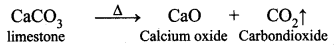(ii) When a magnesium ribbon is burnt in air, it is an oxidation reaction.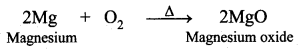Question 2.
What is a combustion reaction?
A combustion reaction is one in which the reactant rapidly combines with oxygen to form one or more oxides and energy (heat). So in combustion reactions, one of the reactants must be oxygen.

Question 3.
Which of the following is a combustion?

1. Digestion of food
2. Rusting of iron.

1. Digestion of food : Not a combustion reaction, because it is a endothermic process and where energy is utilized.
2. Rusting of iron : Combustion reaction.

Question 4.
When the lead powder is added to copper chloride solution, a displacement reaction occurs and solid copper is formed.
(i) Write the equation for the reaction.
(ii) Why does the displacement reaction occur?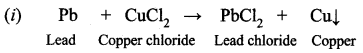(ii) Copper is less reactive than lead. So lead has displaced copper from copper chloride. It is a displacement reaction.

Question 5.
What happens when lead nitrate reacts with potassium iodide solution?
Lead nitrate solution when reacts with a potassium iodide solution, a deep yellow precipitate of Pbl2 is formed.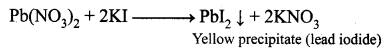Question 6.
Differentiate Exothermic reaction and Endothermic reaction.

 Exothermic reaction Endothermic reaction 1. The chemical reactions which take place with the evolution of heat energy are called exothermic reactions. 1. The chemical reactions which take place with the absorption of heat energy a called endothermic reactions. 2. e.g. All combustion reactions are exothermic. 2. e.g. Dissolution of glucose is the endothermic reaction. 3. N2 + 3H2 → 2NH3 + Heat 3. 2NH3 + Heat → N2 + 3H2

Question 7.
Why the study of reaction rate is important?
Faster the reaction, more will be the amount of the product in a specified time. So, the rate of a reaction is important for a chemist for designing a process to get a good yield of a product. Rate of reaction is also important for a food processor who hopes to slow down the reactions that cause food to spoil.

Question 8.
What is meant by decomposition reaction? Give an example.
A single compound breaks down to produce two or more substances. Such type of reaction is called decomposition reaction.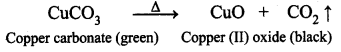Question 9.
Give reason.
(a) Granulated Zinc reacts faster with 2M HCl than 1M HCl.
Reason: As the concentration of the reactant increases the rate of the reaction increases.

(b) Food kept at room temperature spoils faster than that kept in the refrigerator.
Reason: In the refrigerator the temperature is lower than room temperature, so the reaction rate is less.

Question 10.
What is meant by double displacement reaction? Give an example.
A double decomposition reaction is a reaction in which the exchange of ions between two reactants occur leading to the formation of two different products.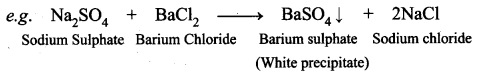Question 11.
Why the toothpastes are generally basic in nature?
The pH of the saliva is usually between 6.5 to 7.5, when the pH of the mouth saliva falls below 5.5 the enamel coating of our teeth calcium phosphate gets weathered. That is why the tooth pastes are generally basic in nature.

Question 12.
What happens to food containing fat and oil kept open for a long time?
When food containing fat and oil is left as such for a long time, it becomes stale. The stale food develops bad taste and foul smell. This is very common in curd and cheese, particularly in summer. Oils and fats are slowly oxidised to certain foul – smelling compounds.

Question 13.
Define the rate of a chemical reaction.
The rate of a chemical reaction is defined as the change in concentration of any one of the reactants or product per unit time.
Rate =−d[A]dt.

Question 14.
Define catalyst.
A substance which alters the rate of a reaction without undergoing any change in mass and composition is known as a catalyst.

Question 15.
What happens during a chemical reaction?

• In a chemical reaction, the atoms of the reacting molecules or elements are rearranged to form new molecules.
• Old chemical bonds between atoms are broken and new chemical bonds are formed.
• Bond breaking absorbs energy whereas bond formation releases energy.

Question 16.
What; is a balanced chemical equation?
A balanced chemical equation is the simplified representation of a chemical reaction which describes the chemical composition, the physical state of the reactants and the products, and the reaction conditions.

Question 17.
What are the main classes of decomposition reactions?
There are three main classes of decomposition reactions. They are,

• Thermal decomposition reactions
• Electrolytic decomposition reactions
• Photo decomposition reactions

Question 18.
What are thermal decomposition reactions?
In a thermal decomposition reaction, the reactant is decomposed by applying heat.
For example, on heating mercury (II) oxide is decomposed into mercury metal and oxygen gas.
2HgO(s)⟶Δ2Hg(l)+O2(g).

Question 19.
What are Electrolytic decomposition reactions?
In this type of reaction, the reactant is decomposed by applying electricity.
For example, decomposition of sodium chloride occurs on passing electric current through its aqueous solution.
2NaCl(aq)⟶ Electricity 2Na(s)+Cl2(g).

Question 20.
What is the photodecomposition reaction?
In this type of reaction, the reactant is decomposed by applying light.
For example, when silver bromide is exposed to light, it breaks down into silver metal and bromine gas.
2AgBr(s)⟶Light2Ag(s)+Br2(g).

Question 21.
What is Metathesis reaction?
The ion of one compound is replaced by the ion of another compound. Ions of identical charges are only interchanged, i.e. a cation can be replaced by another cation. This reaction is called metathesis reaction.

Question 22.
What is a precipitation reaction?
When aqueous solution of two compounds are mixed, if they react to form an insoluble compound and a soluble compound, then it is called precipitation reaction.
e.g„ Pb(NO3)2(aq)+2KI(aq)⟶PbI2(s)↓+2KNO3(aq).

Question 23.
How will you distinguish between combination and decomposition reactions?

 Combination Reactions Decomposition Reactions One or more reactants combine to form a single product A single reactant is decomposed to form one or more products Energy is released Energy is absorbed Elements or compounds may be the reactants The single compound is the reactant

Question 24.
What is a neutralization reaction?
It is another type of displacement reaction in which the acid reacts with the base to form a salt and water. It is called neutralization reaction as both acid and base neutralizes each other.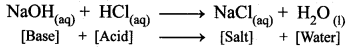Question 25.
What is Combustion reaction?
A combustion reaction is one in which the reactant rapidly combines with oxygen to form one or more oxides and energy (heat).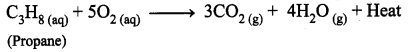Question 26.
What are reversible and irreversible reactions?
Reversible reaction : A reversible reaction is a reaction that can be reversed, i.e., the products can be converted back to the reactants.
e.g., PCl5(g)⇌PCl3(g)+Cl2(g)
Irreversible reaction: The reaction that cannot be reversed is called irreversible reaction. The irreversible reactions are unidirectional.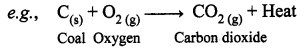Question 27.
Why is the reaction rate important?
Faster the reaction, more will be the amount of the product in a specified time. So, the rate of a reaction is important for a chemist for designing a process to get a good yield of a product. Rate of reaction is also important for a food processor who hopes to slow down the reactions that cause food to spoil.

Question 1.
Suggest a reason for each observation given below.
(i) In fireworks, powdered Mg is used rather than Mg ribbon.
Powdered Mg will have larger surface area than Mg ribbon and the rate of the reaction increases.

(ii) Zn and dil. H2SO4 react much more quickly when a few drops of CuSO4 solution are added.
When few drops of CuSO4 are added to the solution containing Zn and dil. H2SO4, the rate of the reaction increases, because CuSO4 acts as catalyst.

(iii) The reaction between MgCO3 and dil. HCl speeds up when some con. HCl is added. As the concentration of the reactant increases, the rate of the reaction increases.

Question 2.
Observe the given chemical change and answer the following: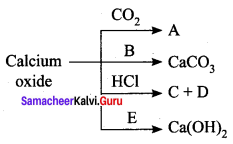1. Identify ‘A’ and ‘B’.
2. Write the commercial name of calcium hydroxide.
3. Identify products ‘C’ and ‘D’ when HCl is allowed to oxide react with calcium oxide.
4. Say whether calcium oxide is acidic or basic.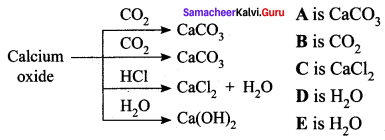1. A is calcium carbonate. B is carbon-di-oxide.
2. Slaked lime is the commercial name of calcium hydroxide.
3. The products C and D are calcium chloride (CaCl2) and water (H2O).
4. Calcium oxide is basic in nature.

Question 3.
Take copper nitrate in a test tube and heat it over the flame.

1. What is the colour of cupric nitrate?
2. What do you observe?
3. Name the type of reaction that takes place.
4. Write the balanced equation.

1. The colour of cupric nitrate is Blue.
2. When cupric nitrate is heated in a test tube, we can observe the evolution of reddish-brown gas (NO2) Nitrogen dioxide.
3. The reaction takes place is a decomposition reaction.
4.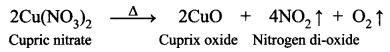Question 4.
Redox reactions are reactions during which electron transfer takes place. Here magnesium atom transfers two electrons one each to the two chlorine atoms.
(i) What are the products of this reaction?
(ii) Write the balanced equation for the complete reaction.
(iii) Which element is being oxidized?
(iv) Which element is being reduced?
(v) Write the reduction part of the reaction.
(i) Magnesium atom is converted to Mg2+ ion. Two chlorine atoms are converted to 2Cl ions. So the products are Mg2+ ion and 2Cl ions.
(ii)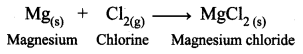(iii) Mg atom is being oxidised by donating 2 electrons.
(iv) Cl2 molecule is being reduced by accepting 2 electrons.
(v) Cl2 + 2e → 2Cl. It is a reduction reaction in which gain of e take place.

Question 5.
Take Cu(NO3)2 in a test tube and heat it over the flame.
(i) What is the colour of Cu(NO3)2?
Blue

(ii) What do you observe?
Evolution of reddish-brown gas.

(iii) Name the type of reaction that takes place.
Decomposition.

(iv) Write the balanced equation.
Cu(NO3)2 → 2CuO + 4NO2 + O2

Question 6.
Sodium hydroxide and hydrochloric acid react as shown in this equation.
NaOH(aq)+HCl(aq)⟶NaCl(aq)+H2O(l)
(i) Which type of chemical reaction is this?
(ii) The reaction is exothermic. Explain what that means.
(iii) Differentiate exothermic reaction and endothermic reaction.
(iv) What happens to the temperature of the solution as the chemicals react?
(i) It is a neutralisation reaction.
(ii) An exothermic reaction is a chemical reaction in which the evolution of heat energy takes place.
(iii)

 Exothermic reaction Endothermic reaction 1. The reaction In which heat energy is evolved is known as an exothermic process. 1. The reaction in which heat energy is absorbed is known as an endothermic process. 2. N2 + 3H2 → 2NH3 + Heat 2. 2NH3 + Heat → N2 + 3H2

(iv) When NaOH reacts with HCl to give NaCl and water, heat is evolved. So the solution’s temperature increases.

VIII. HOT Questions.

Question 1.
Equal lengths of magnesium ribbons are taken in test tubes A and B. Hydrochloric acid is added to test tube A, while acetic acid is added to test tube B. The amount and concentration taken for both the acids are same. In which test tube does the reaction occur more vigorously and why?
In the test tube, the reaction occurs more vigorously.
Comparing hydrochloric acid and acetic acid, HCl (hydrochloric acid) is a strong acid and more reactive whereas acetic acid is a weak organic acid and less reactive.
Mg+2HCl⟶ Fast reaction MgCl2+H2↑.

Question 2.
Classify the following reactions based on the rate of the reactions as very fast or instantaneous slow and moderate reactions.
(a) AgNO3(aq) + NaCl(aq) → AgCl ↓ + NaNO3(aq)
Very fast reaction (or) Instantaneous reaction.

(b) 2 Na(s) + 2H2O(l) → 2NaOH(aq) + H2(g)
Very fast reaction.

(c) Rusting of iron.
Very slow reaction.

(d) Inversion of cane sugar into Glucose and fructose.
Moderately slow reaction.

(e) Fermentation of sugar into alcohol.
Very slow reaction.

Question 3.
What is the chemical reaction taken place in the tarnishing of silver anklet?
The lustrous white colour of the silver anklet slowly changes into a slightly black colour. It is due to the formation of silver sulphide (Ag2S) as a result of the reaction between silver and hydrogen sulphide in the air.

Question 4.
Why toothpaste are basic in nature?
The white enamel coating of our teeth is calcium phosphate, the hardest substance in our body. It does not dissolve in water. If the pH of mouth falls below 5.5, the enamel gets corroded. Toothpaste is generally basic and used for cleaning the teeth can neutralize the excess acid and prevent tooth decay.

Question 5.
Why the solution of slaked lime is used for whitewashing?
A solution of slaked lime is used for whitewashing walls. Calcium hydroxide reacts slowly with the carbon dioxide in the air to form a thin layer of calcium carbonate on the walls. Calcium carbonate is formed after two to three days of whitewashing and gives a shiny finish to the walls. It is interesting to note that the chemical formula for marble is also CaCO3.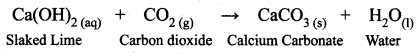Question 6.
Complete the following reactions.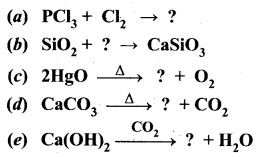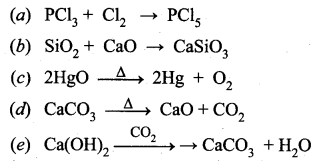Question 7.
Let us consider the following two reactions.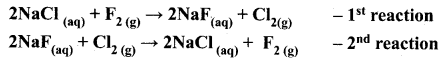Which reaction will not occur. Why?
The first reaction involves the displacement of chlorine from NaCl, by fluorine. In the second reaction, chlorine displaces fluorine from NaF. Out of these two, the second reaction will not occur. Because fluorine is more active than chlorine and occupies the upper position in the periodic table. So, in displacement reactions, the activity of the elements and their relative position in the periodic table are the key factors to determine the feasibility of the reactions. More active elements readily displace less active elements from their aqueous solutions.

Question 8.
Which of the metals displaces hydrogen gas from hydrochloric acid? Silver or zinc. Give the chemical equation of the reaction and justify your answer.
Zinc displaces hydrogen gas from hydrochloric, acid.
Zinc is more reactive than silver.
Zn + 2HCl → ZnCl2 + H2 ↑.

Question 9.
Foods kept at room temperature spoils faster than that kept in the refrigerator. Why?
Food kept at room temperature spoils faster than that kept in the refrigerator. In the refrigerator, the temperature is lower than the room temperature and hence the reaction rate is less.

Question 10.
How will you enhance the rate of decomposition of potassium chlorate?
On heating potassium chlorate, it decomposes into potassium chloride and oxygen gas, but at a slower rate. If manganese dioxide is added, it increases the reaction rate.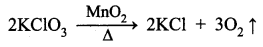Here MnO2 is the catalyst. Therefore the addition of MnO2 enhances the rate of decomposition of potassium chlorate.

Question 11.
Powdered calcium carbonate reacts more readily with hydrochloric acid than marble chips. Why?
Powdering of the reactants increases the surface area and more energy is available on the collision of the reactant particles. Thus, the reaction rate is increased. Hence powdered calcium carbonate reacts more readily with hydrochloric acid than marble chips.

### Samacheer Kalvi 10th Science Types of Chemical Reactions Additional Problems Solved

Question 1.
The [OH] ion concentration of a solution is 1.0 × 10-8 M. What is the pH of the solution?
Solution:
The concentration of hydroxide ion = [OH] = 1.0 × 10-8 M.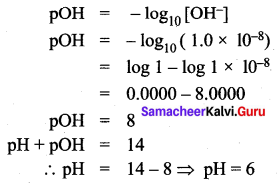Question 2.
The hydrogen ion concentration of a solution is 1.0 × 10-9 m. What is the pH of the solution? Find out whether the given solution is acidic, basic or neutral.
Solution: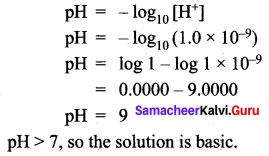Question 3.
The hydroxide ion concentration of a solution is 0.001 m. What is the pH of the solution?
Solution: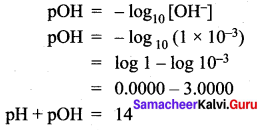pH = 14 – pOH
pH = 14 – 3 = 11
pH = 11.

Question 4.
The hydroxide ion concentration of a solution is 1.0 × 10-9 m. What is the pH of the solution?
Solution: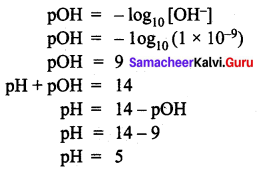Question 5.
The hydrogen ion concentration of a solution is 1 × 10-4 m. Calculate the pH and pOH of that solution.
Solution: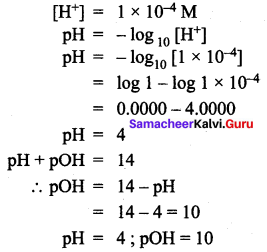Question 6.
Calculate the pH of sodium hydroxide solution having the concentration of OH 0.01 mL-1.
Solution: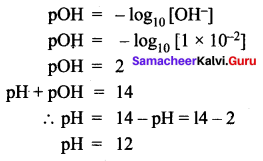## How to Prepare using Samacheer Kalvi 10th Science Chapter 10 Types of Chemical Reactions Notes PDF?

Students must prepare for the upcoming exams from Samacheer Kalvi 10th Science Chapter 10 Types of Chemical Reactions Notes PDF by following certain essential steps which are provided below.

• Use Samacheer Kalvi 10th Science Chapter 10 Types of Chemical Reactions notes by paying attention to facts and ideas.
• Pay attention to the important topics
• Refer TN Board books as well as the books recommended.
• Correctly follow the notes to reduce the number of questions being answered in the exam incorrectly
• Highlight and explain the concepts in details.

## Frequently Asked Questions on Samacheer Kalvi 10th Science Chapter 10 Types of Chemical Reactions Notes

#### How to use Samacheer Kalvi 10th Science Chapter 10 Types of Chemical Reactions Notes for preparation??

Read TN Board thoroughly, make separate notes for points you forget, formulae, reactions, diagrams. Highlight important points in the book itself and make use of the space provided in the margin to jot down other important points on the same topic from different sources.

#### How to make notes for Samacheer Kalvi 10th Science Chapter 10 Types of Chemical Reactions exam?

Read from hand-made notes prepared after understanding concepts, refrain from replicating from the textbook. Use highlighters for important points. Revise from these notes regularly and formulate your own tricks, shortcuts and mnemonics, mappings etc.
Share: